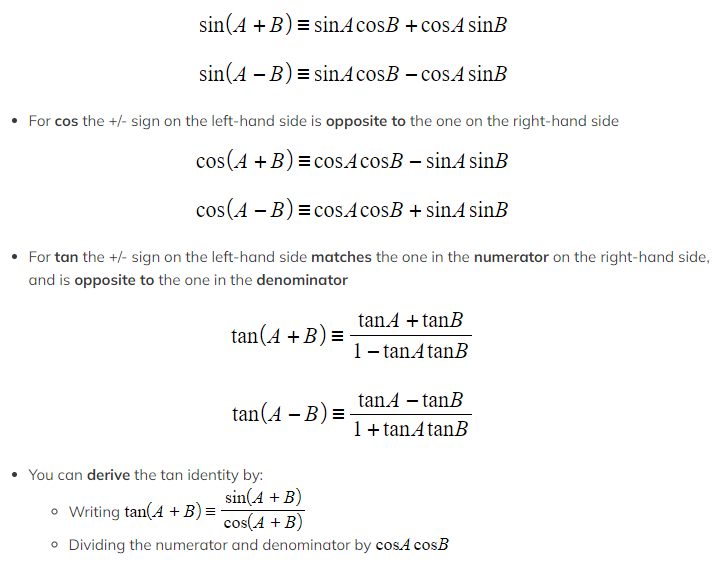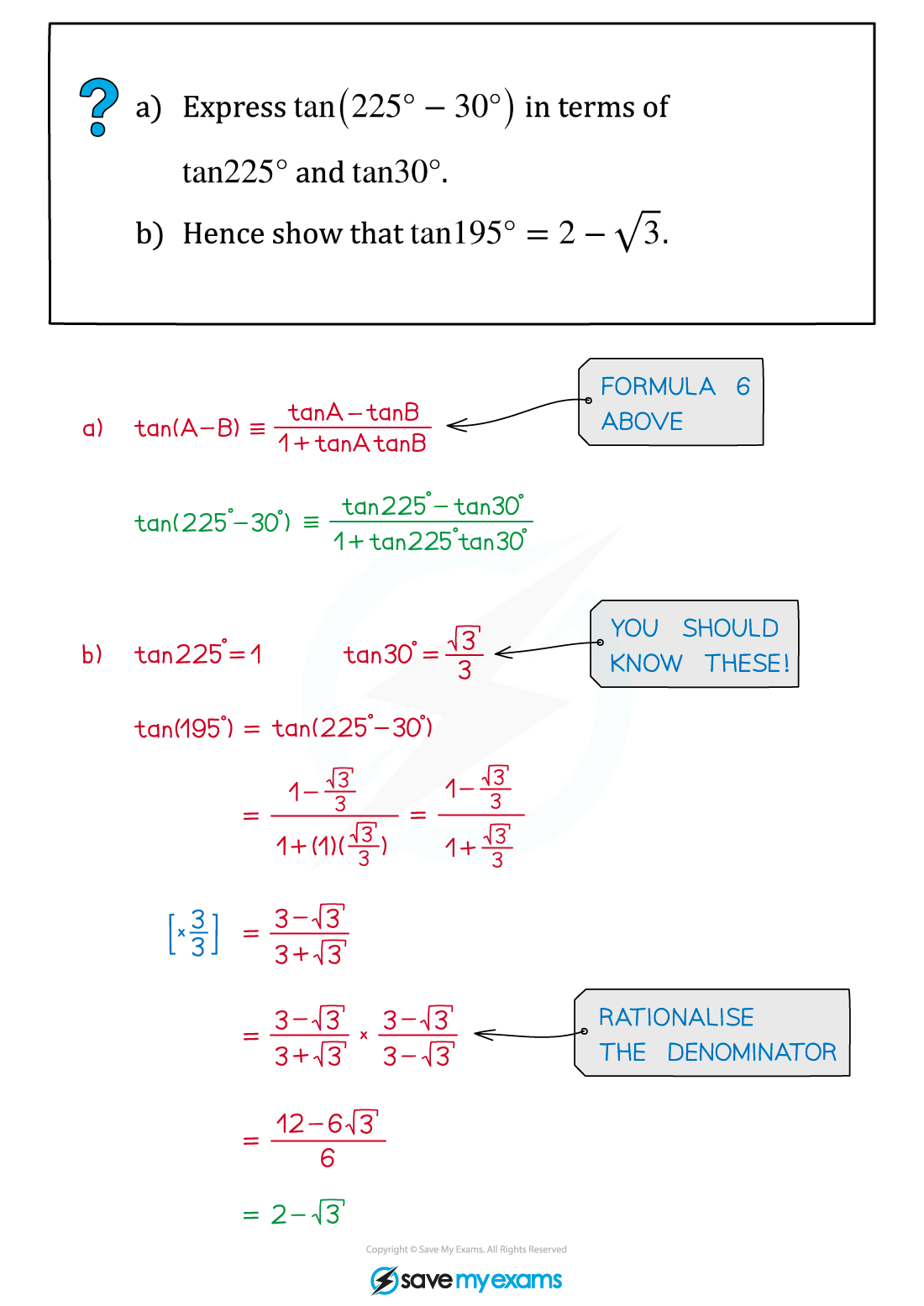# AQA A Level Maths: Pure复习笔记5.6.1 Compound Angle Formulae

### Compound Angle Formulae

#### What are the compound angle formulae?

• There are six compound angle formulae (also known as addition formulae), two each for sin, cos and tan:
• For sin the +/- sign on the left-hand side matches the one on the right-hand side

####Exam Tip

• All these formulae are in the formulae booklet – you don't have to memorise them.

#### Worked Example